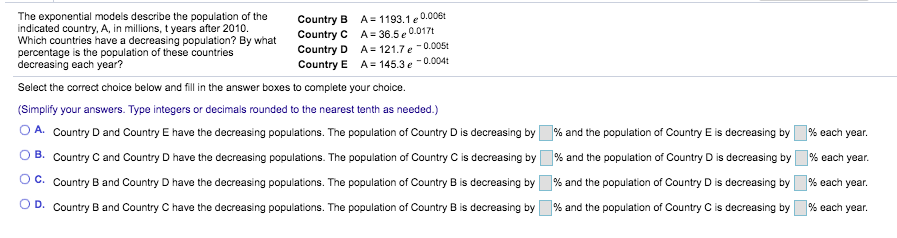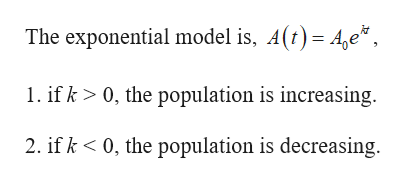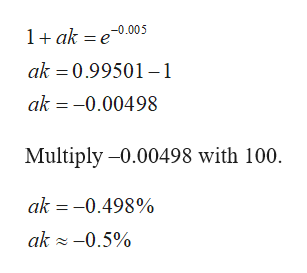# The exponential models describe the population of theindicated country, A, in millions, t years after 2010.Which countries have a decreasing population? By whatpercentage is the population of these countriesdecreasing each year?0.006A 1193.1 eA 36.5 e0.017tCountry BCountry CCountry DCountry EA 121.7e-0.005tA = 145.3 e0.004tSelect the correct choice below and fill in the answer boxes to complete your choice.(Simplify your answers. Type integers or decimals rounded to the nearest tenth as needed.)O A. Country D and Country E have the decreasing populations. The population of Country D is decreasing byB. Country C and Country D have the decreasing populations. The population of Country C is decreasing by% and the population of Country D is decreasing by% each year.% and the population of Country E is decreasing by% each yearO C. Country B and Country D have the decreasing populations. The population of Country B is decreasing by% and the population of Country D is decreasing by% each year.D. Country B and Country C have the decreasing populations. The population of Country B is decreasing by% each year.% and the population of Country C is decreasing by

Questionhelp_outlineImage TranscriptioncloseThe exponential models describe the population of the indicated country, A, in millions, t years after 2010. Which countries have a decreasing population? By what percentage is the population of these countries decreasing each year? 0.006 A 1193.1 e A 36.5 e0.017t Country B Country C Country D Country E A 121.7e-0.005t A = 145.3 e0.004t Select the correct choice below and fill in the answer boxes to complete your choice. (Simplify your answers. Type integers or decimals rounded to the nearest tenth as needed.) O A. Country D and Country E have the decreasing populations. The population of Country D is decreasing by B. Country C and Country D have the decreasing populations. The population of Country C is decreasing by% and the population of Country D is decreasing by % each year. % and the population of Country E is decreasing by % each year O C. Country B and Country D have the decreasing populations. The population of Country B is decreasing by % and the population of Country D is decreasing by % each year. D. Country B and Country C have the decreasing populations. The population of Country B is decreasing by % each year. % and the population of Country C is decreasing by fullscreen
check_circleExpert Solution
Step 1

Result used:help_outlineImage TranscriptioncloseThe exponential model is, A(t) Ae 1. ifk> 0, the population is increasing. 0, the population is decreasing 2. ifk fullscreen
Step 2

From the given four countries, it is observed that the countries D and E only have the negative k values.

Thus, Country D and Country E have the decreasing populations.

Step 3

Use the formula (1 +ak) to convert the continuous compound growth to annual ...help_outlineImage Transcriptionclose1ak e0.005 ak 0.99501-1 ak -0.00498 Multiply -0.00498 with 100 ak -0.498% ak -0.5% fullscreen

### Want to see the full answer?

See Solution

#### Want to see this answer and more?

Solutions are written by subject experts who are available 24/7. Questions are typically answered within 1 hour*

See Solution
*Response times may vary by subject and question
Tagged in

### Other